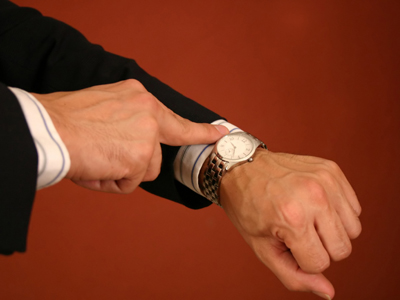What is 28,800 seconds in hours? Find out in this quiz.

# Time (Difficult)

In this 11-plus Maths quiz, you'll do a few calculations involving different measures of time. Spend some time reading the questions carefully and see if you can get 10 out of 10. You should do the previous quizzes before you do this quiz.

Just as we have words to describe large amounts of time, we also have words for very small amounts of time. You might think that a second is the tiniest amount of time, but you'd be wrong. There are milliseconds, microseconds and even nanoseconds. Do you know what the smallest measurable unit of time is called? It's called Planck time, named after the German physicist Max Planck.

Anyway, you won't be learning about such extremes of times in your 11-plus, so let's concentrate on this quiz.

1.
How many revolutions (complete turns) does the minute hand of a clock turn through in 1 minute?
3,600 revolutions
60 revolutions
1 revolution
None
The minute hand of a clock turns through one complete revolution in 1 hour. In one minute it turns through 1/60th of a revolution because there are 60 minutes in an hour
2.
How many seconds are there in a day?
860,400 seconds
864 seconds
86,400 seconds
8,640 seconds
There are 60 seconds in 1 minute, 60 minutes in 1 hour, and 24 hours in a day: 60 × 60 × 24 = 86,400 seconds
3.
Peter's watch is running fast and shows the time as 23:47. How many minutes does Peter's watch have to be wound back to show the correct time of 22:18?
It has to be wound back 29 minutes
It has to be wound back 90 minutes
It has to be wound back 89 minutes
It has to be wound back 98 minutes
Find out how fast it is running: this is the number of minutes that it has to be wound back. From 22:18 to 23:00 = 42 minutes. From 23:00 to 23:47 is 47 minutes, so the watch needs to be wound back 47 + 42 = 89 minutes
4.
John starts shift work in New Town at 19:55. One day he left home at 18:05 and travelled by bus to the station: he got to the station at 18:47. The train journey took 37 minutes to get to New Town, and it took him a further 18 minutes of walking to get to work. What time did John get to work, and by how many minutes was he late or early?
He got to work at 7:42 pm, and he was early by 13 minutes
He got to work at 7:42 pm, and he was late by 13 minutes
He got to work at 7:42 am, and he was early by 13 minutes
He got to work at 20:42 pm, and he was early by 13 minutes
From home to the station took 18:47 - 18:05 = 42 minutes. The train journey took 37 minutes, so he got to New Town at 18:47 + 37 minutes = 19:24. He got to work at 19:24 + 18 minutes = 19:42. He was 19:55 - 19:42 = 13 minutes early
5.
How many revolutions (complete turns) does the second hand of a clock turn through in 1 day?
360 revolutions
60 revolutions
14,440 revolutions
1,440 revolutions
It goes round once for every minute. There are 60 minutes in an hour, so that's 1 × 60 = 60 revolutions an hour. There are are 24 hours in a day, so there are 24 × 60 = 1,440 revolutions in a day
6.
How many days are there in a decade which has three leap years?
3,666 days
3,647 days
3,653 days
3,650 days
A decade is ten years. There are 365 days in a year, so 10 × 365 = 3,650 days. Each leap year adds an extra day; therefore, the total number of days = 3,650 + 3 = 3,653 days
7.
What is 28,800 seconds in hours?
8 hours
80 hours
0.8 hours
6 hours
There are 60 seconds in 1 minute, so in 28,800 seconds there are 28,800 ÷ 60 = 480 minutes. There are 60 minutes in 1 hour, so in 480 minutes there are 480 ÷ 60 = 8 hours. Each time you divide, you are finding how many 'lots' of one thing there are in another thing. There are 8 hours in 480 minutes because 8 'lots' of 60 equals 480. You could keep on adding 60 to itself until you got to 480: it's the same thing, but division is quicker
8.
How many months are there in a decade?
1,200 months
100 months
12 months
120 months
A decade is 10 years. There are 12 months in a year, so that's 10 × 12 = 120 months
9.
The bus leaves at 18:47 and arrives at 19:23. How many minutes does the journey take?
37 minutes
36 minutes
38 minutes
136 minutes
18:47 to 19:00 is 13 minutes. 19:00 to 19:23 is 23 minutes. The total time = 13 + 23 = 36 minutes
10.
How many half hour periods are there in a day?
24 half hour periods
48 half hour periods
96 half hour periods
12 half hour periods
There are 24 hours in a day. Each hour has 2 half hour periods, so there are 24 × 2 = 48 half hour periods
Author:  Frank Evans